Browse by Topic
Related Topics

# Mathematica and The American Mathematical Monthly’s “Problems and Solutions” Section

The “Problems and Solutions” section of The American Mathematical Monthly journal has always been a source of interesting problems to keep me entertained. Their solutions often require ingenuity. The problems in the October issue were no exception.

I always analyze and explore these problems in Mathematica. Being a kernel developer, I see whether Mathematica can indeed find a solution. This last issue has challenging problems, and it was particularly gratifying to observe that Mathematica could solve them right out of the box. So here are my solutions to three of the paraphrased problems:

Problem 11457, by M. L. Glasser: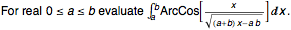Solution in Mathematica: Relaxing assumptions on a and b to avoid degenerate cases: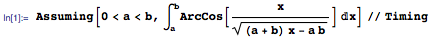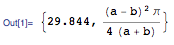Problem 11456, by R. Mortini: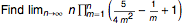Solution in Mathematica: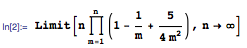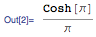Problem 11449, by M. Bataille:Solution in Mathematica: As the expression is left unchanged by the variable’s rescaling, and as c is positive, let us set c==1.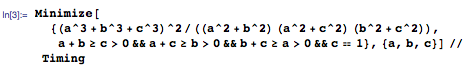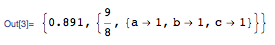Hence the minimal value of 9/8 is attained for a==b==c.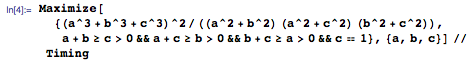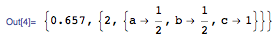Because the expression is left invariant by interchanging any of the variables, the maximum value of 2 is attained for a==b==c/2 or a==c==b/2 or b==c==a/2.

Mathematica can help solve practically any computation problem. Its thousands of functions are detailed in the online Documentation Center, with many tutorials and examples.